### N.9 Ex­pla­na­tion of the band gaps

Chap­ter 6.21 showed that the spec­tra of the elec­trons of solids have band gaps; en­ergy ranges for which there are no quan­tum states for the elec­trons. These band gaps were qual­i­ta­tively ex­plained as the rem­nants of the dis­crete elec­tron en­ergy states of the in­di­vid­ual atoms. These dis­crete en­ergy states spread out when mul­ti­ple atoms start in­ter­act­ing, but not nec­es­sar­ily enough to com­pletely re­move the gaps.

How­ever, if you start from the free-elec­tron gas point of view, it is much less clear why and when ad­di­tion of just a bit of crys­tal po­ten­tial would sud­denly pop up band gaps out of noth­ing. If you are cu­ri­ous, this note is for you.

To un­der­stand what is go­ing on, the Kro­nig & Pen­ney model will be used. The crys­tal is again taken to be one-di­men­sion­al. The po­ten­tial con­sists again of a se­quence of straight dips, as was shown in green in 6.22. The dips rep­re­sent the at­trac­tion of the atoms on the in­di­vid­ual atomic elec­trons. How­ever, to al­low an eas­ier com­par­i­son with the free-elec­tron gas so­lu­tions, this time the dips will taken far less deep than be­fore. Think of it as a model for a metal, where the outer elec­trons are only very weakly bound to their atomic cores.

For these shal­lower atomic dips, and for a crys­tal con­sist­ing of very many atoms, the en­ergy lev­els are as shown to the left in fig­ure N.1. Note that for the higher en­er­gies, this is gen­er­ally speak­ing very sim­i­lar to the en­ergy lev­els for the free-elec­tron spec­trum shown to the left. That should be ex­pected; why would the shal­low po­ten­tial en­ergy dips have much of an ef­fect when the ki­netic en­ergy of the elec­tron con­sid­ered is very large? But even for high en­ergy lev­els, there are still oc­ca­sional thin gaps. At these gaps, the elec­tron ve­loc­ity plunges to zero. Why are these gaps there?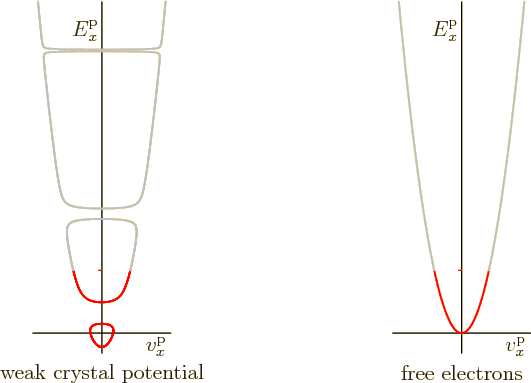To qual­i­ta­tively un­der­stand what is go­ing on, from here on it will be as­sumed that the pe­ri­odic crys­tal con­sists of just 12 atoms, (rather than, say, a mil­lion). Math­e­mat­i­cally, af­ter twelve atoms, the quan­tum wave func­tion be­comes the same as it was ini­tially and the so­lu­tion re­peats. You may think of the twelve atoms as phys­i­cally be­ing arranged in a ring shape.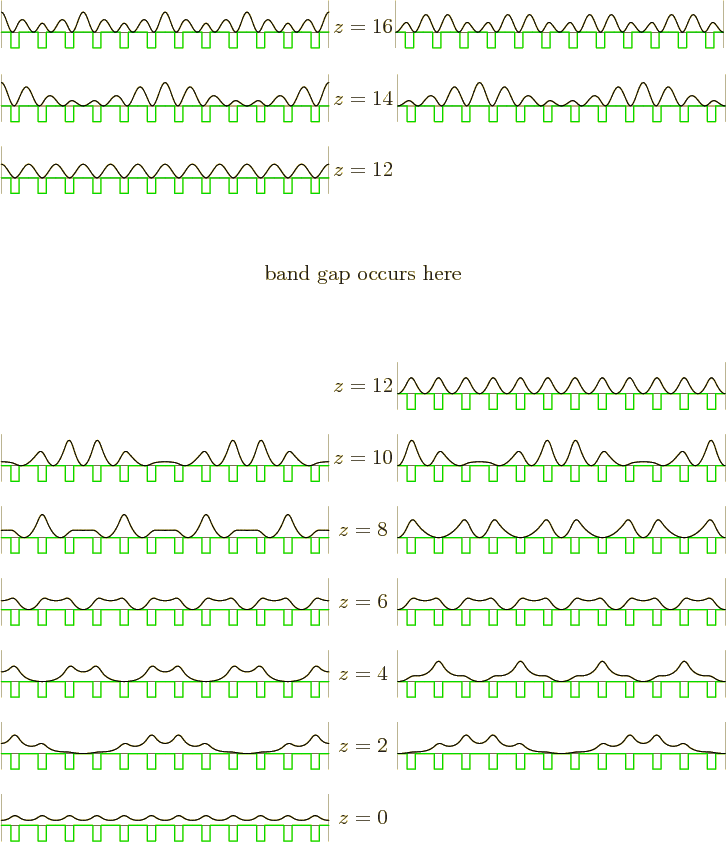To make things eas­ier to un­der­stand, it is also de­sir­able to switch from the com­plex Bloch wave wave func­tions to the equiv­a­lent real ones. These real wave func­tions may be found as the real and imag­i­nary parts of the Bloch waves. That is eas­i­est for the free-elec­tron gas, where the Bloch waves are sim­ply com­plex ex­po­nen­tials; the Euler iden­tity says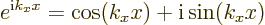So for the free-elec­tron gas, the real wave func­tions are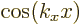and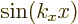, ig­nor­ing an unim­por­tant nor­mal­iza­tion con­stant. As be­fore, the wave num­ber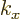is a mea­sure of the “crys­tal mo­men­tum”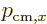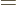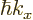, which is turn re­lated to the elec­tron ve­loc­ity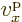through the en­ergy.

For the Kro­nig-Pen­ney model, the real wave func­tions are more com­plex than sim­ple sines and cosines, but can be found the same way.

Note that nor­mally there are two dif­fer­ent wave func­tions for each value of the wave num­ber. The one ex­cep­tion is for the ground state of low­est en­ergy whereis zero. In terms of the free elec­tron gas,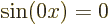. Zero is not a valid wave func­tion. Re­mem­ber that the square mag­ni­tude of a wave func­tion gives the prob­a­bil­ity of find­ing the elec­tron. So if a wave func­tion would be zero, there would not be any chance of find­ing the elec­tron any­where. So there would be no elec­tron.

For the free-elec­tron gas, that leaves as only ground state wave func­tion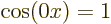times some con­stant. That is just a con­stant. And since the square of a con­stant is still a con­stant, that means that the prob­a­bil­ity of find­ing the elec­tron is the same every­where in the pe­riod.

For the Kro­nig-Pen­ney case, the sit­u­a­tion is a less sim­ple. Con­sider first the ground state, shown in the pic­ture at the bot­tom of fig­ure N.2. (In fig­ure N.2 the height of a wave func­tion pic­ture il­lus­trates the rel­a­tive amount of en­ergy of that wave func­tion. So the ground state pic­ture is at the bot­tom.) The square mag­ni­tude of the ground state wave func­tion, shown as the black line, is no longer a con­stant. It is higher than av­er­age at the dips in the po­ten­tial, at the atoms. It is lower than av­er­age in be­tween atoms. So the elec­tron is some­what more likely to be found near an atom that at­tracts it than in be­tween atoms. The elec­tron re­duces its po­ten­tial en­ergy that way. But it can­not do this with­out limit; if the elec­tron is only found at the atoms, the re­duced un­cer­tainty in po­si­tion in­creases the ki­netic en­ergy more than the po­ten­tial en­ergy is low­ered. The best com­pro­mise is given by the black line at the bot­tom of fig­ure N.2.

To un­der­stand the en­ergy states above the ground state, a key con­cept of the gen­eral math­e­mat­i­cal prop­er­ties of real one-di­men­sion­al wave func­tions is needed: The more zero cross­ings in the wave func­tion, the higher the en­ergy. Qual­i­ta­tively, the rea­son is not that hard to un­der­stand. The more zero cross­ings, the more wildly the wave func­tion swings back and for­ward be­tween pos­i­tive and neg­a­tive val­ues, rais­ing the ki­netic en­ergy. In fig­ure N.2 the num­ber of zero cross­ings is listed as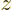. Note that by squar­ing the wave func­tions, the zero cross­ings be­come touch­ing zero, not cross­ing it.

Note fur­ther that only even num­bers of zero cross­ingsap­pear. A pe­ri­odic wave func­tion must re­turn to the same sign at the end of the pe­riod as at the start, and that is only pos­si­ble if the num­ber of zero cross­ings is even.

Note next that in al­most all cases, there are two dif­fer­ent wave func­tions of the same en­ergy at a given num­ber of zero cross­ings. That is be­cause if you have one wave func­tion at a given, you can sim­ply shift it over by one atomic cell, and you have an­other wave func­tion of the same en­ergy. This sec­ond wave func­tion is al­most al­ways a dif­fer­ent one. In par­tic­u­lar, it can only be the same wave func­tion if theze­ros are still in the same place. But if you have 12 atomic cells and, say,8 ze­ros, then some of the 12 atomic cells must have ze­ros and other ones not. So the shifted wave func­tion can­not pos­si­bly have all its ze­ros in the same place. So the shifted wave func­tion is a sec­ond, dif­fer­ent, wave func­tion of the same en­ergy. So there are two dif­fer­ent wave func­tions with the sameand en­ergy.

The only way this can pos­si­bly fail, and does, is if each atomic cell has the same num­ber of ze­ros as its next neigh­bor. So every atomic cell must have the same num­ber of ze­ros in it.

That, then, is why it is pos­si­ble at all that there is only one wave func­tion in the ground state. In the ground state there are no ze­ros, so every atomic cell has the same num­ber, none. In­deed, look­ing closer, in the ground state the wave func­tion is iden­ti­cal in every atomic cell. Math­e­mat­i­cally, for0, the ex­po­nen­tial part of the Bloch wave is just a triv­ial con­stant, mak­ing the com­plete Bloch wave the same for all atomic cells. So shift­ing the wave func­tion over one atomic cell gives you back the ex­act same thing.

The next pos­si­bil­ity that the shifted wave func­tion does not give a dif­fer­ent one oc­curs when every atomic cell has one zero cross­ing. For a crys­tal of 12 atomic cells, that re­quires that there are12 zero cross­ings. This hap­pens when the wavenum­ber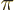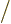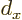whereis the atomic cell size. Then the ex­po­nen­tial part of the Bloch wave func­tion in any atomic cell is iden­ti­cal to that in the next atomic cell ex­cept for a mere mi­nus sign. So the shifted wave func­tion is only dif­fer­ent by a mi­nus sign. This means it is phys­i­cally equiv­a­lent to the orig­i­nal one. Not a sep­a­rate wave func­tion.

But even if shift­ing the wave func­tion does not give you a sec­ond one, still there must be two dif­fer­ent eigen­func­tions for each even num­ber of ze­rosgreater than zero. In par­tic­u­lar, the wave func­tions in fig­ure N.2 were ob­tained in two ways. For the wave func­tions in the left-hand col­umn, it was as­sumed that the de­riv­a­tive of the wave func­tion is zero at the start of the pe­riod (like it is for thefree-elec­tron gas so­lu­tions). For the wave func­tions in the right-hand col­umn, it was as­sumed that the wave func­tion it­self is zero at the start of the pe­riod (like it is for thefree-elec­tron gas so­lu­tions). These are two dif­fer­ent so­lu­tions; they can­not be equiv­a­lent be­cause the right-hand wave func­tion has a zero at the start of the pe­riod, but the left-hand one does not.

For one, this ex­plains why for the ground state where0, there is no right-hand wave func­tion. If you start out with a zero cross­ing, you must have at least one of them. It also ex­plains why a wave func­tion in the right col­umn is not just the wave func­tion in the left col­umn shifted by an atomic cell. The two wave func­tions were sep­a­rately com­puted. In al­most all cases, the right-hand wave func­tion is then a com­bi­na­tion of the shifted and un­shifted left-hand wave func­tions, the com­bi­na­tion that is zero at the start of the pe­riod. In about half the cases, that turns out to be the left wave func­tion shifted by a quar­ter pe­riod, in the other half of the cases it is just all dif­fer­ent. The en­ergy of the two so­lu­tions is still the same.

But for the spe­cial case of12 ze­ros for the 12 atomic cells, fig­ure N.2 shows that the left and right wave func­tions are phys­i­cally fun­da­men­tally dif­fer­ent. In the left-hand wave func­tion, the elec­tron is most likely to be found in the re­gion of high po­ten­tial en­ergy be­tween atoms. All the peaks in wave func­tion are there. In the right-hand wave func­tion, the elec­tron is most likely to be found in the re­gion of low po­ten­tial en­ergy at the atoms. The peaks are there. That means that the left hand wave func­tion has a lot more po­ten­tial en­ergy than the right-hand one. So the two wave func­tions do not have the same en­ergy in this case. We have a band gap when the num­ber of ze­ros is ex­actly the same as the num­ber of atoms.

Sim­i­larly there will be a band gap at24, where there are two zero cross­ings in each atomic cell, etcetera. The band gaps oc­cur at whole mul­ti­ples of the num­ber of atomic cells. And there are 12 en­ergy states in each band. For a phys­i­cally re­al­is­tic num­ber of atomic cells, call it a mil­lion in­stead of 12, there are a mil­lion en­ergy states be­tween band gaps, ef­fec­tively form­ing a con­tin­uum band be­tween the gaps.

One thing that may still be counter-in­tu­itive is why the right-hand12 wave func­tion has higher, rather than much lower en­ergy than the10 ones. In par­tic­u­lar, the peaks in the right-hand wave func­tion at12 are all per­fectly aligned with the atomic lo­ca­tions. That greatly re­duces the po­ten­tial en­ergy. But in the10 case the peaks are not aligned with the atoms. While the10 case has some ad­van­tage in ki­netic en­ergy with less zero cross­ings, that ad­van­tage is small. That would not be able to ex­plain it if the right-hand12 would re­ally have a big ad­van­tage in po­ten­tial en­ergy over the10 states.

To see why it is pos­si­ble, look more closely at the10 case in fig­ure N.2. It is true that a sig­nif­i­cant frac­tion of the peaks in wave func­tion are in be­tween atoms in­stead of on top of atoms. How­ever, the physics mod­u­lates the height of the peaks so that the big peaks are the ones on top of the atoms, and the small peaks the ones in be­tween atoms. That still has the ef­fect that the elec­tron is most likely found at an atom, still greatly re­duc­ing the po­ten­tial en­ergy. That elim­i­nates the ap­par­ent ad­van­tage of the right-hand12 state in po­ten­tial en­ergy.

Also note that for10, the num­ber of ze­ros is still close to the num­ber of atoms. So the dis­tance be­tween peaks is still al­most the same as the dis­tance be­tween atoms, (es­pe­cially for a mil­lion atoms in­stead of 12). So if, say, a peak is pretty much on top of an atom, the neigh­bor­ing peaks are too. There­fore the mod­u­la­tion of peak am­pli­tudes can be done in a way that slowly varies along the length of the crys­tal. So it does not add a big amount to the ki­netic en­ergy.

How­ever, be­cause of the mod­u­la­tion, the10 wave func­tions do give up al­most all their small ki­netic en­ergy ad­van­tage com­pared to the right-hand12 case. That means that the two en­er­gies be­come very close to­gether. Since the change in en­ergy is a mea­sure of the elec­tron prop­a­ga­tion ve­loc­ity, that ve­loc­ity plunges to zero. Which is ex­actly what you see in fig­ure N.1.

Sim­i­larly for the14 wave func­tions, the peaks are mod­u­lated so that the elec­tron is most likely to be found in be­tween atoms, just like for the left-hand12 wave func­tion. So the14 wave func­tions have about the same po­ten­tial en­ergy as the left-hand12 one. And be­cause the12 wave func­tion is so ef­fec­tive in rais­ing its po­ten­tial en­ergy, you would ex­pect that the en­ergy dif­fer­ence with the14 case would be rel­a­tively small, pro­duc­ing small elec­tron ve­loc­ity. And that is in­deed what hap­pens.

So the only fi­nite en­ergy gaps oc­cur when the num­ber of ze­ros is a whole mul­ti­ple of the num­ber of atoms. And the gap is be­tween the two states with that num­ber of ze­ros.

And be­tween the states im­me­di­ately above and be­low the gaps, the en­ergy dif­fer­ence is even smaller than else­where in the band. That makes the elec­tron ve­loc­ityzero at the edges of the bands

Since the wave func­tions at the edges of the bands have zero prop­a­ga­tion ve­loc­ity, elec­trons in these states can­not move through the crys­tal. Now an im­plicit re­sult of the analy­sis above is that for these states, a whole mul­ti­ple of the Bloch wave length must equal dou­ble the atomic spac­ing. The Bloch ex­po­nen­tial can change sign go­ing from one atomic cell to the next, then re­turn to the orig­i­nal sign at the next cell, but noth­ing more. If you train a beam of elec­trons with a wave length like that onto the crys­tal, the beam can­not prop­a­gate and will be to­tally re­flected. That is in fact a key re­sult of the Bragg re­flec­tion the­ory of wave me­chan­ics, (10.16) in chap­ter 10.7.2. Thus Bragg the­ory can pro­vide an in­tu­itive jus­ti­fi­ca­tion for some of the fea­tures of the band struc­ture.

If you want to see math­e­mat­i­cally that the prop­a­ga­tion ve­loc­ity is in­deed zero at the band gaps, and you know lin­ear al­ge­bra, you can find the de­riva­tion in {D.84}. That also ex­plains how the wave func­tion fig­ures in fig­ure N.2 were made.